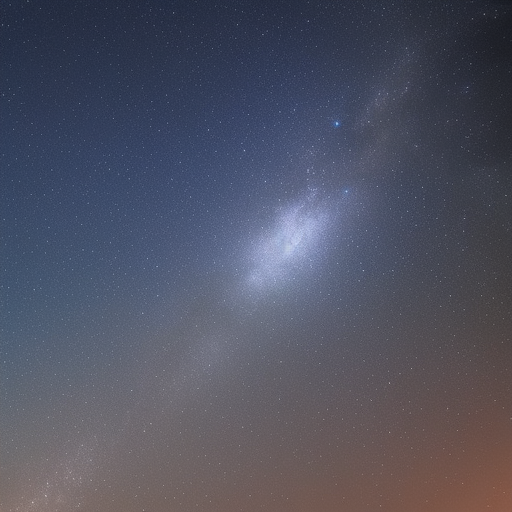David's Blog

# Using OpenCV in Python A Comprehensive Guide

By David Li on 2023-11-24T14:04:38.000Z# Using OpenCV in Python: A Comprehensive Guide

OpenCV (Open Source Computer Vision Library) is a powerful and widely-used library for computer vision, image processing, and machine learning applications. It is designed to work with various programming languages, including Python. This article will provide a comprehensive guide on how to use OpenCV in Python, covering installation, basic operations, image processing techniques, and more.

## Installation

To get started with OpenCV in Python, you need to install the `opencv- package. You can do this using the following command:

``pip install opencv-python``

If you need the additional modules that are not included in the main package, you can install the `opencv-contrib- package:

``pip install opencv-contrib-python``

## Reading, Displaying, and Saving Images

To read an image using OpenCV, you can use the `imread()` function. This function takes the image file path as an argument and returns the image in a NumPy array format.

``````import cv2

To display the image, you can use the `imshow()` function. This function takes two arguments: the name of the window and the image itself.

``````cv2.imshow("Image", image)
cv2.waitKey(0)
cv2.destroyAllWindows()``````

The `waitKey()` function waits for a keyboard event, and the `destroyAllWindows()` function closes all the open windows.

To save an image, you can use the `imwrite()` function. This function takes the file path and the image as arguments.

``cv2.imwrite("output.jpg", image)``

## Basic Image Operations

### Grayscale Conversion

To convert an image to grayscale, you can use the `cvtColor()` function with the `COLOR_BGR2GRAY` flag.

``gray_image = cv2.cvtColor(image, cv2.COLOR_BGR2GRAY)``

### Resizing Images

To resize an image, you can use the `resize()` function. This function takes the image and the desired dimensions as arguments.

``resized_image = cv2.resize(image, (width, height))``

### Rotating Images

To rotate an image, you can use the `getRotationMatrix2D()` and `warpAffine()` functions. The first function computes the rotation matrix, and the second applies the matrix to the image.

``````(height, width) = image.shape[:2]
center = (width // 2, height // 2)

matrix = cv2.getRotationMatrix2D(center, angle, scale)
rotated_image = cv2.warpAffine(image, matrix, (width, height))``````

## Image Processing Techniques

### Image Thresholding

To apply a binary threshold to an image, you can use the `threshold()` function. This function takes the image, the threshold value, the maximum value, and the threshold type as arguments.

``_, thresholded_image = cv2.threshold(gray_image, threshold_value, max_value, cv2.THRESH_BINARY)``

### Edge Detection

To detect edges in an image, you can use the `Canny()` function. This function takes the image and the lower and upper thresholds for the edges as arguments.

``edges = cv2.Canny(image, lower_threshold, upper_threshold)``

### Blurring Images

To blur an image, you can use various techniques, such as Gaussian blur, median blur, or bilateral blur. For example, to apply a Gaussian blur, you can use the `GaussianBlur()` function.

``blurred_image = cv2.GaussianBlur(image, (kernel_size, kernel_size), sigma)``

## Face Detection Using Haar Cascades

To detect faces in an image, you can use the pre-trained Haar Cascade classifiers. First, you need to load the classifier using the `CascadeClassifier()` function.

``face_cascade = cv2.CascadeClassifier("haarcascade_frontalface_default.xml")``

Next, you can use the `detectMultiScale()` function to detect faces in the image. This function takes the image, the scale factor, and the minimum number of neighbors as arguments.

``````faces = face_cascade.detectMultiScale(gray_image, scaleFactor=1.1, minNeighbors=5)

## Draw rectangles around the detected faces
for (x, y, w, h) in faces:
cv2.rectangle(image, (x, y), (x + w, y + h), (255, 0, 0), 2)``````

## Conclusion

This article provided an overview of using OpenCV in Python, covering installation, basic operations, image processing techniques, and face detection using Haar Cascades. OpenCV is a powerful library that can be used for various computer vision and image processing tasks. By mastering its functions, you can build sophisticated applications, such as object tracking, image segmentation, and even deep learning-based object recognition.

For more information on OpenCV and its functions, visit the official documentation.

© Copyright 2023 by Astro Tech Blog. Built with ♥ by FriendlyUser. Last updated on 2023-12-07.